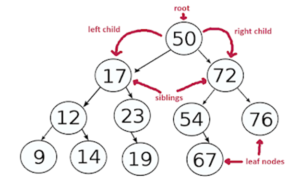# Tree Traversal – Inorder, Preorder and Postorder

Here you will learn about tree traversal with program example.

Tree is a subset of Graph data structure where the number of edges are exactly one less than the number of vertices (nodes). We can call any graph a tree if it does not have any cycle (closed loop).

Tree traversal refers to the process of visiting each node of the tree at least once. Unlike basic linear data structures like arrays, linked list, stack and queue where there was only one way of traversing, trees can be traversed in different ways.

We can categorize the tree traversal into two categories:

2. Depth-first Traversal

## Depth-first Traversal

In Depth-first traversal, the direction of traversal is from top to bottom. As the name suggests, we traverse to the depth of the tree starting from the root node of the tree. Based on the way of traversal, we have these three types of traversals:

• In-order Traversal
• Pre-order Traversal
• Post-order Traversal

These three types of traversal are usually performed on binary trees. Binary tree is a special type of tree in which a parent node can have at most two child nodes. Binary Search Tree (BST) is a special binary tree where every smaller value is on the left of the parent node and every greater value is on the right of the parent node.Let’s see these traversals in detail.

### In-order Traversal

As the name suggest, in in-order traversal we traverse the tree in the order that we humans normally use. We first visit the left subtree after that we visit the parent of the left subtree and then we visit the right subtree. When we use in-order traversal on a Binary Search Tree (BST), we get the data in sorted order (ascending order).

Algorithm for In-order Traversal

1. Check that the current node is not null, if null return to the previous call.
2. Make recursive call to the in-order function for traversing the left subtree.
3. Print the value of the current node.
4. Make recursive call to the in-order function for traversing the right subtree.

### Pre-order Traversal

Pre-order traversal is the type of traversal in which we first visit the root node of the tree. After the root node, we visit the left subtree and finally we visit the right subtree. The Pre-order traversal is mainly used to create a copy of the existing tree. It is also used in the evaluation of expressions, for example: we can create a prefix form of an expression using the pre-order traversal.

Algorithm for Pre-order Traversal

1. Check that the current node is not null, if null return to the previous call.
2. Print the value of the current node.
3. Make recursive call to the pre-order function for traversing the left subtree.
4. Make recursive call to the pre-order function for traversing the right subtree.

### Post-order Traversal

In Post-order Traversal, the tree is traversed in the order of left->right->root. That means we first visit the left subtree after that we visit the right subtree and finally we visit the root node. The Post-order traversal is used during deletion of the tree. It is suitable for deletion operation because it first deletes the child nodes then it deletes the parent node.

Algorithm for Post-order Traversal

1. Check that the current node is not null, if null return to the previous call.
2. Make recursive call to the post-order function for traversing the left subtree.
3. Make recursive call to the post-order function for traversing the right subtree.
4. Print the value of the current node.